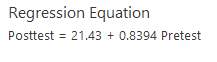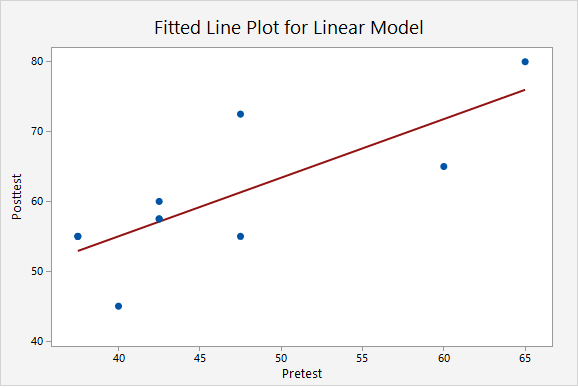# 3.4.3.2 - Example: Interpreting Output

This example uses the "CAOSExam" dataset available from http://www.lock5stat.com/datapage.html.

CAOS stands for Comprehensive Assessment of Outcomes in a First Statistics course. It is a measure of students' statistical reasoning skills. Here we have data from 10 students who took the CAOS at the beginning (pre-test) and end (post-test) of a statistics course.

Research question: How can we use students' pre-test scores to predict their post-test scores?

Minitab Express was used to construct a simple linear regression model. The two pieces of output that we are going to interpret here are the regression equation and the scatterplot containing the regression line.Let's work through a few common questions.

What is the regression model?

The "regression model" refers to the regression equation. This is $$\widehat {posttest}=21.43 + 0.8394(Pretest)$$

Identify and interpret the slope.

The slope is 0.8394. For every one point increase in a student's pre-test score, their predicted post-test score increases by 0.8394 points.

Identify and interpret the y-intercept.

The y-intercept is 21.43. A student with a pre-test score of 0 would have a predicted post-test score of 21.43.  However, in this scenario, we should not actually use this model to predict the post-test score of someone who scored 0 on the pre-test because that would be extrapolation. This model should only be used to predict the post-test score of students from a comparable population whose pre-test scores were between approximately 35 and 65.

One student scored 60 on the pre-test and 65 on the post-test. Calculate and interpret that student's residual.

This student's observed x value was 60 and their observed y value was 65.

$$e=y- \widehat y$$

We have y.  We can compute $$\widehat y$$ using the x value and regression equation that we have.

$$\widehat y = 21.43 + 0.8394(60) = 71.794$$

$$e=65-71.794=-6.794$$

This student's residual is -6.794. They scored 6.794 points lower on the post-test than we predicted given their pre-test score.